﻿ Genetic Algorithm for Task Allocation and Path Planning of Multi-robot System

### Genetic Algorithm for Task Allocation and Path Planning of Multi-robot System

Zhenping Li, Xueting Li

Journal of Mathematical Sciences and Applications

## Genetic Algorithm for Task Allocation and Path Planning of Multi-robot System

Zhenping Li1,, Xueting Li1

1School of Information, Beijing Wuzi University, Beijing, China

### Abstract

Based on the consideration of the collision, the task allocation and path planning of multi-robot system are studied. With robots’ the longest travel time as a restrictive condition and the total cost minimum as the objective function, the integer programming model is established; In order to avoid robots colliding in the process of walking, a collision penalty term is introduced and a genetic algorithm with collision detection is designed. Using the genetic algorithm, the optimal solution of task allocation and path planning can be found effectively, the paths can avoid the robots collision. Finally, the model and algorithm are verified by a specific example.

• Zhenping Li, Xueting Li. Genetic Algorithm for Task Allocation and Path Planning of Multi-robot System. Journal of Mathematical Sciences and Applications. Vol. 4, No. 1, 2016, pp 34-38. http://pubs.sciepub.com/jmsa/4/1/6
• Li, Zhenping, and Xueting Li. "Genetic Algorithm for Task Allocation and Path Planning of Multi-robot System." Journal of Mathematical Sciences and Applications 4.1 (2016): 34-38.
• Li, Z. , & Li, X. (2016). Genetic Algorithm for Task Allocation and Path Planning of Multi-robot System. Journal of Mathematical Sciences and Applications, 4(1), 34-38.
• Li, Zhenping, and Xueting Li. "Genetic Algorithm for Task Allocation and Path Planning of Multi-robot System." Journal of Mathematical Sciences and Applications 4, no. 1 (2016): 34-38.

 Import into BibTeX Import into EndNote Import into RefMan Import into RefWorks

1
Prev Next

### 1. Introduction

Multi-robot system refers to a number of robots to complete lots of tasks coordinately in a specific environment. Multi-robot system is mainly divided into centralized system and non-centralized system, while the difference between them is whether using the central processor to control the system. This paper focuses on the centralized system, which uses the central processor to realize the task allocation and path planning of robots.

Multi-robot system has been successfully used in many practical works, such as cargo handling in dangerous goods warehouse, data collection in extreme environment, or environmental monitoring and so on. Li  studied the task allocation problem of multi-robot in smart warehouse of “cargo-to-person” picking mode and gave the effective polity to complete cargo handling; Liu  studied the path planning of multi-robot based on dynamic priority; In remote operation, Suzuki T  studied multi-robot system completed the task of the detection; Based on avoiding collision, Yamashita  put up the method for path planning of multi-robot cooperative transportation of large objects; Silva  proposed a genetic algorithm to solve the problem of multi-robot cooperation sohoban. “Robogas” project team members use multi-robot system to solve the problem of gas leakage of the plant's monitoring points. Later based on this project, the simulation using 3 robots, finished 90 position monitoring, Kelin Jose  proposed A* algorithm to solve the path planning of robots and genetic algorithm to solve tasks allocating of 90 points monitoring. In the process of monitoring 90 points, Kelin Jose set in advance using three robots, rather than using optimal robots through calculation analysis, making the cost is not necessarily the lowest. On the other hand, a collision for robots did not implement the punishment mechanism.

Robots’ collision can cause damage, prolong working time, and even cause the system to be paralyzed, so the number of robots collision among paths should be reduced as much as possible. In order to minimize the number of robots collision, the task allocation and path planning problem of multi-robot system is studied in this paper. Based on considering the fixed cost of robots, the penalty of number of collisions is introduced, and a genetic algorithm is designed to solve the task allocation and path planning problem of multi-robot system, the optimal usage of robots and the minimal cost can be obtained by the algorithm.

### 2. Problem Description and Mathematical Model

2.1. Problem Description

Multi-robot system for environmental monitoring is used in a factory ,The factory has m homogeneous robots to monitor n monitoring stations, each monitoring station needs to be detected once a day. Each robot full of electricity can run limited distance continuously, and the detected time of each monitoring point is given. When not performing detecting tasks, the robots are charged at the platform; when a group of detection tasks needed to be performed, all robots run from the starting platform at the same time to complete respective detection tasks along the planned path. Robots should avoid mutual collision in the process of walking, and return to the starting platform after completing the detection tasks. In the daily work, each robot only needs to complete the detection tasks assigned to it, each detection task is just completed by one robot. Due to the longer time required for the robot to charge, the total travel distance (time) of robots are limited. The fixed cost of calling each robot and unit distance cost of driving each robot are given, how to assign n detection tasks to m robots and how to plan the path of each robot so as to minimize the total cost of detecting tasks every day?

2.2. Mathematical Model

In order to establish the mathematical model, the following symbols are defined: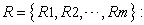A set of robots that can be used;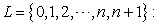Represents the set of starting platform and monitoring points,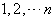represent the monitoring points, 0 is the starting platform, and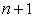can be seen as a copy of the starting platform;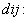Represents the distance from point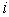to point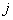of robots,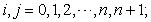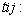Represents the robot’s driving time from pointto point,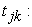Represents the time that pointis monitored by robot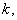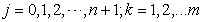;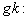Represents the fixed cost of calling robot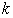,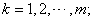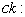Represents the driving unit distance cost of robot,The decision variables are defined as follows: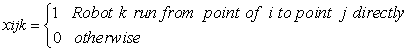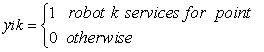Without considering collision, to minimize the total cost, the task allocation and path planning can be formulated into an integer linear programming model as follows: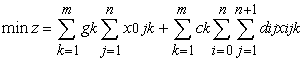(1)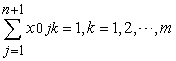(2)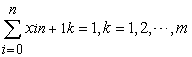(3)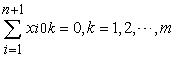(4)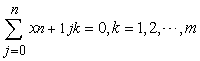(5)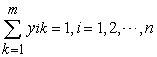(6)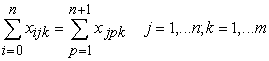(7)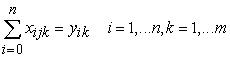(8)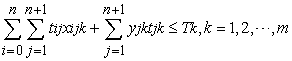(9)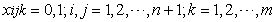(10)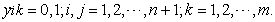(11)

(1) Represents to minimize the total cost;

(2) Represents each robot must start from platform 0;

(5) Represents no robot can leave from point n+1;

(6) Represents each monitoring point must be serviced by one robot;

(7) Represents each robot arrived at monitoring point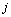must leave from the monitoring point;

(8) Represents a robot serviced for a monitoring point must have reached the monitoring point;

(9) Represents the constraint of maximum driving time for each robot;

(10), (11) represent the constraint of variable value

The above integer linear programming model does not consider the collisions of robots’ paths. In practice, the collisions of robots should be avoided as far as possible in the path planning of robots. To reduce the collisions of robots’ paths, a penalty term is added into the objective function with the number of collisions between robots’ paths, and then we will design a genetic algorithm with collision detection to solve the problem.

### 3. Genetic Algorithm with Collision Detection

In order to describe the principle of algorithm simply and clearly, the genetic algorithm combined with a specific example this section will be introduced. Figure 3.1 shows a plant layout. In order to prevent gas leakage of the plant, each possible points with gas leakage must be monitored by robots. Each point only needs to be monitored once and all points must be monitored every day. The Red Square in Figure 3.1 represents the starting platform of m robots, the gray area represents the building and robots cannot walk across it. Serial number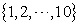represents 10 points to be monitored, each of which is fixed to a coordinate. Robot can only run in the direction of North and South, East and West, and robots speed is 1 m/min with known paths. Based on avoiding collision, how to allocate robots’ tasks and plan their paths so as to minimize the total cost?Download asVeiw figureFigures index
Figure 3.1. Plant layout
3.1. Coding and Decoding Rules of Genetic Algorithm

(1) Coding rule

Natural number coding method is adopted to represent the structure of the solution, with 0 indicating the starting platform and natural numbers indicating point numbers. For example, (0 3 4 6 0 7 5 9 1 0 2 10 8 0) represents 3 robots to complete the monitoring tasks and the monitoring paths are 0-3-4-6-0, 0-7-5-9-1-0, 0-2-10-8-0. In order to remove 0, the solution is expressed as the sequence of point numbers, which is (3 4 6 7 5 9 1 2 10 8).

(2) Decoding rule

Due to omitting the starting platform during the encoding, we arrange robots to service for monitoring points as little as possible in the decoding. For example, a coding is (3 4 6 7 9 5 1 1 2 8). Assigning the first robot departs from the platform 0 to service for the monitoring point 3, then considering various constraints decide whether it can serve for point 4, if the condition is satisfied, the point 4 will be serviced by the first robot, then taking into account the continuous working time of the robot, determine whether it can serve point 6, if the condition is satisfied, the point 6 will be serviced by the first robot. Also considering a variety of constraints, determine whether point 7 can be serviced by the first robot, we find that the condition is not satisfied, so the robot returns to the starting platform. By now, we find the scheme about the path planning and task assignment of the first robot, which is 0-3-4-6-0. Continuously, it’s time to assign the paths and tasks of the second robot. It still departs from the platform 0 and services point 7 firstly. Arrange the tasks and paths of the second robot as the same principles as those of the first robot. We can find the scheme of the second robot 0-7-5-9-1-0. Then the third scheme is 0-2-10-8-0. Now, all monitoring points have been arranged for each robot, which forms a feasible solution.

3.2. Collision Detection and Penalty

(1) The starting platform is expressed with number 0, so the number sequence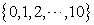has 11 points, each robot departs from the platform to perform its tasks and returns to the platform. There are a total of 121 paths, which can be expressed by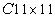. Each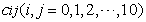represents the path from pointto point. For example,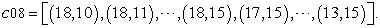(2) The paths of all robots are detected in pairs. For example, one individual in the population is 0-7-0-3-8-0-5-4-0-2-1-0-6-0-10-9-0.The first robot returns to the starting platform after monitoring point 7, and the second robot returns to the starting platform after monitoring point 8. Similarly, until the sixth robot monitors its points, all tasks have been completed. When tasks are performed, it is assumed that 6 robots are simultaneously starting from the platform, so the paths of all robots are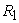is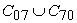and the path of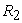is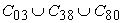. That is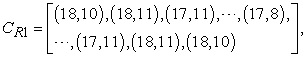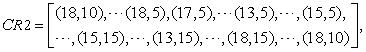Comparing the paths ofand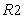, their identical coordinates are (18, 10) and (18, 11), and there exist two times collisions in the process of coming and going. The coming and going ofare expressed by one and two, so the time arrived at (18, 10) can be expressed as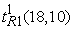and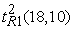.In the same way: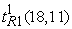and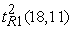,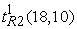and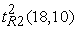,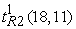and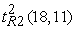. Compare the operation of different robots to the same coordinates 8 times. If two robots reach the same coordinates at the same time, the collision is happened. For example,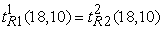it represents that the time ofarriving at (18, 10) is the same as, soandare collided. Finally, accumulate their collisions and return. If a collision happens on two robots, then return to 1, otherwise 0.

(3) Similarly, detect whether there exist collisions between two robots. If there exists, record it and accumulate their total collisions.

(4) Define the collision penalty terms by the collisions of all robots in one individual. With total number of collisions in each individual, measuring their respective intensity of punishment, the total collision number can be represents as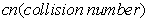. The punishment coefficient is defined as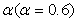.The objective function increased with penalty term is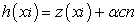, where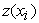represents the total cost corresponding to all paths of the individual.

3.3. Basic Steps of Genetic Algorithm

(1) Initial population

The initial population is obtained by the method of random generation, and the Q arrangement of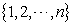is generated, and the Q is the population size.

(2) Fitness function

Firstly, the decoding of each individual is a feasible solution, and the total cost of the solution is obtained. Then, get the value of objective function increased with collision penalty, Furthermore, calculate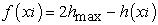, where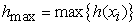. Finally, calculate the fitness function value according to the following formula: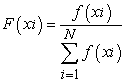(3) Selection

Roulette selection method is utilized to select individual, and preferentially selected individuals are called the parents with larger fitness value that contribute to the population at the generation. Meanwhile, the best retention method is introduced to ensure the final result at the termination of genetic algorithm that the appeared individuals in all ages possess the largest fitness function value.

(4) Crossover

In this paper, we use the partial matching crossover, which is to determine the crossover point in the gene sequence of the father, and the partial sequence of the crossing points to form a mapping relationship and then to exchange the corresponding, so as to form a new generation of individual; This does not set the crossover parameters, the first in different individuals to cross, reflecting the diversity of the nature of the population.

(5) Mutation

By using swap mutation method, two swap positions are randomly generated in the selected individual, the genes of which are transferred, so as to form a new individual; in this paper, we do not set mutated parameters, but to analyze mutated populations and determine whether to operate partial mutation, and concrete rules are as follows:

1) If the quality of mutated population is better than last generation, it does not operate mutation and directly step into the next generation;

2) Otherwise, carry out mutated operation on the population whose fitness value is lower than average. After mutating, if the whole quality of population is better than before, we do the mutated operation and directly step into the next generation; otherwise, it is still not improved after several times mutations, then directly eliminating the individual and replacing it with the best individual before. If the quality of the replaced population is still not as good as previous generation, we will operate mutations on the population whose fitness value is lower than average, repeat this step until the whole quality of the population is better than the previous generation, we step into next generation.

(6) Termination

When the evolution generation is G, stop calculation and output the optimal solution obtained in the process of calculation.

### 4. Simulation Result

A factory has 10 points leaking gas and required monitoring daily, there are 10 robots starting from the same platform numbered 0 to service for 10 points. Each point needs to be monitored for 10 minutes. Each robot charged full can work for 10 hours continuously , the fixed cost of calling each robot is 60 Yuan and the cost of each robot running one meter is 2 Yuan. The layout of a factory is shown in Figure 1, where the gray area represents impractical region. Table 4.1 shows the distance between starting platform and monitoring points and Table 4.2 shows the time of robots driving between the platforms and monitoring points.

#### Table 4.2. the time of robots driving between platform and monitoring points (unit: hour)

Running the genetic algorithm coded by Matlab, we can get the total number of collisions and obtain an objective function of genetic algorithm with penalty items. Setting the size of population is 50 and the maximum number of evolution generation is 100. In the contest of Windows 7 (g4, 2G, 32-bit operating system), running the procedure for 30 times, we obtain the average driving time is 12.4 seconds and average driving distance is 1772 meters, making the average driving cost is 3852.7 Yuan with 5 robots. One of the convergent process about genetic algorithm is showed in figure 4.1. We can obtain the optimal solution in about 40 iterations. .In this paper, with the best reservation mechanism, the optimal solution will not change too much when we continue iterating after obtaining it. Figure 4.2 shows the paths of 5 robots respectively: 0-3-0; 0-9-10-0; 0-1-2-0; 0-5-4-0; 0-6-7-8-0.Download asVeiw figureFigures index
Figure 4.1. a curve of convergent process about genetic algorithmDownload asVeiw figureFigures index
Figure 4.2. the driving paths of robots

From Figure 4.2, we can see the paths of the 5 robots, of which double arrow indicates backtrack. At the same time departing from platform, there is no collision occurring in the process of robots completing tasks. Even with the same driving points in the process of return, there is no collision due to the times robots driving to the same points are different with the same speed.

### 5. Conclusion

Based on avoiding the collisions of robots, genetic algorithm is used to solve the problem of task allocation and path planning in this paper. That is, in constraint of the longest running time of robots, using the optimal number of robots to complete monitoring tasks and making the lowest total cost. However, there are still many deficiencies in this paper. In order to simplify problems, we assume that all robots depart at the same time. In fact, we can adjusted the times robots depart to reduce collisions in practice. Meanwhile, this paper does not consider the waiting mechanism in the driving process of robots, which is also a strategy to avoid collisions. In the premise of sharing information and avoid collisions, we will consider using the optimal number of robots and planning the optimal path for robots to minimize the total cost in next study.

### Acknowledgements

This work was supported by National Natural Science Foundation of China (11131009, 71540028),And Major Research Project of Beijing Wuzi University, High lever scientific research project of Beijing Wuzi University (GJB20164005).The Funding Project of Beijing high level innovation and entrepreneurship program teaching teacher. Funding project for Beijing key Laboratory of Intelligent Logistics System (NO: BZ0211); Funding project for Beijing intelligent logistics Collaborative Innovation Center and a major research project of Beijing Wuzi University.

### References

  Li, Z. and Li, W. mathematical model and algorithm for the task allocation problem of robots in the smart warehouse [J]. Logistics Technology, 2015(5): 493-502. (In Chinese).In article  Shuang Liu, Dong Sun, Chang Zhu, A dynamic priority based path planning for cooperation of multiple mobile robots in formation forming [J]. ELSEVIER, 2014(30): 589-596.In article  Suzuki T, Sekine T, Fujii T, Asama H and Endo I. Cooperative formation among multiple mobile robot teleportation in inspection task[C]. In: Proceedings of the IEEE conference on decision and control, Sydney, NSW; 2000: 358-63.In article  A. Yamashita, T. Arai, J. Ota, H. Asama, Motion planning of multiple mobile robots for Cooperative manipulation and transportation [J]. IEEE Trans. on Robotics and Automation, 2003(19): 223-237.In article View Article  Y. Wang, C.W. de Silva, A machine-learning approach to multi-robot coordination [J]. Engineering Applications of Artificial Intelligence, 2008(21): 470-484.In article View Article  Kelin Jose, Dilip Kumar Pratihar, Task allocation and collision-free path planning of centralized multi-robot system for industrial plant inspection using heuristic methods [J]. Robotics and Autonomous Systems,2016.In article View Article  Wu,J. Applied analysis and prospect of robots Kiva in the Amazon warehouse [J]. Logistics technology and Application, 2015(10): 159-162.In article